# Tag: braid group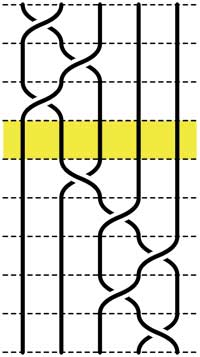Recall that an n-braid consists of n strictly descending elastic strings connecting n inputs at the top (named 1,2,…,n) to n outputs at the bottom (labeled 1,2,…,n) upto isotopy (meaning that we may pull and rearrange the strings in any way possible within 3-dimensional space). We can always change the braid slightly such that we can divide the interval between in- and output in a number of subintervals such that in each of those there is at most one crossing.

n-braids can be multiplied by putting them on top of each other and connecting the outputs of the first braid trivially to the inputs of the second. For example the 5-braid on the left can be written as $B=B_1.B_2$ with $B_1$ the braid on the top 3 subintervals and $B_2$ the braid on the lower 5 subintervals.

In this way (and using our claim that there can be at most 1 crossing in each subinterval) we can write any n-braid as a word in the generators $\sigma_i$ (with $1 \leq i < n$) being the overcrossing between inputs i and i+1. Observe that the undercrossing is then the inverse $\sigma_i^{-1}$. For example, the braid on the left corresponds to the word

$\sigma_1^{-1}.\sigma_2^{-1}.\sigma_1^{-1}.\sigma_2.\sigma_3^{-1}.\sigma_4^{-1}.\sigma_3^{-1}.\sigma_4$

Clearly there are relations among words in the generators. The easiest one we have already used implicitly namely that $\sigma_i.\sigma_i^{-1}$ is the trivial braid. Emil Artin proved in the 1930-ies that all such relations are consequences of two sets of ‘obvious’ relations. The first being commutation relations between crossings when the strings are far enough from each other. That is we have

$\sigma_i . \sigma_j = \sigma_j . \sigma_i$ whenever $|i-j| \geq 2$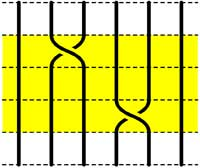=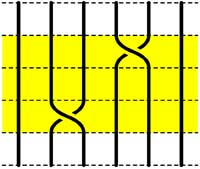The second basic set of relations involves crossings using a common string

$\sigma_i.\sigma_{i+1}.\sigma_i = \sigma_{i+1}.\sigma_i.\sigma_{i+1}$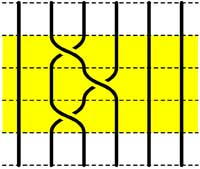=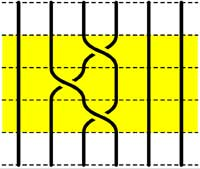Starting with the 5-braid at the top, we can use these relations to reduce it to a simpler form. At each step we have outlined to region where the relations are applied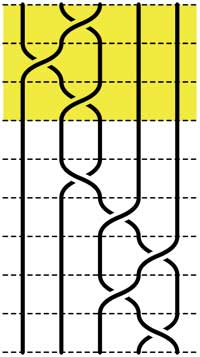=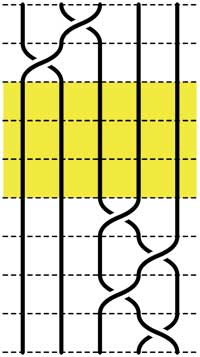=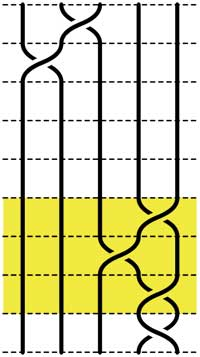=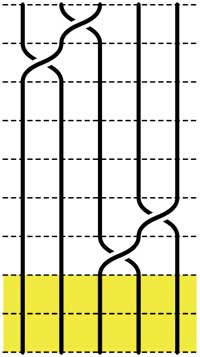These beautiful braid-pictures were produced using the braid-metapost program written by Stijn Symens.

Tracing a string from an input to an output assigns to an n-braid a permutation on n letters. In the above example, the permutation is $~(1,2,4,5,3)$. As this permutation doesn’t change under applying basic reduction, this gives a group-morphism

$\mathbb{B}_n \rightarrow S_n$

from the braid group on n strings $\mathbb{B}_n$ to the symmetric group. We have seen before that the symmetric group $S_n$ has a F-un interpretation as the linear group $GL_n(\mathbb{F}_1)$ over the field with one element. Hence, we can ask whether there is also a F-un interpretation of the n-string braid group and of the above group-morphism.

Kapranov and Smirnov suggest in their paper that the n-string braid group $\mathbb{B}_n \simeq GL_n(\mathbb{F}_1[t])$ is the general linear group over the polynomial ring $\mathbb{F}_1[t]$ over the field with one element and that the evaluation morphism (setting t=0)

$GL_n(\mathbb{F}_1[t]) \rightarrow GL_n(\mathbb{F}1)$ gives the groupmorphism $\mathbb{B}_n \rightarrow S_n$

The rationale behind this analogy is a theorem of Drinfeld‘s saying that over a finite field $\mathbb{F}_q$, the profinite completion of $GL_n(\mathbb{F}_q[t])$ is embedded in the fundamental group of the space of q-polynomials of degree n in much the same way as the n-string braid group $\mathbb{B}_n$ is the fundamental group of the space of complex polynomials of degree n without multiple roots.

And, now that we know the basics of absolute linear algebra, we can give an absolute braid-group representation

$\mathbb{B}_n = GL_n(\mathbb{F}_1[t]) \rightarrow GL_n(\mathbb{F}_{1^n})$

obtained by sending each generator $\sigma_i$ to the matrix over $\mathbb{F}_{1^n}$ (remember that $\mathbb{F}_{1^n} = (\mu_n)^{\bullet}$ where $\mu_n = \langle \epsilon_n \rangle$ are the n-th roots of unity)

$\sigma_i \mapsto \begin{bmatrix} 1_{i-1} & & & \\ & 0 & \epsilon_n & \\ & \epsilon_n^{-1} & 0 & \\ & & & 1_{n-1-i} \end{bmatrix}$

and it is easy to see that these matrices do indeed satisfy Artin’s defining relations for $\mathbb{B}_n$.

It’s been a while, so let’s include a recap : a (transitive) permutation representation of the modular group $\Gamma = PSL_2(\mathbb{Z})$ is determined by the conjugacy class of a cofinite subgroup $\Lambda \subset \Gamma$, or equivalently, to a dessin d’enfant. We have introduced a quiver (aka an oriented graph) which comes from a triangulation of the compactification of $\mathbb{H} / \Lambda$ where $\mathbb{H}$ is the hyperbolic upper half-plane. This quiver is independent of the chosen embedding of the dessin in the Dedeking tessellation. (For more on these terms and constructions, please consult the series Modular subgroups and Dessins d’enfants).

Why are quivers useful? To start, any quiver $Q$ defines a noncommutative algebra, the path algebra $\mathbb{C} Q$, which has as a $\mathbb{C}$-basis all oriented paths in the quiver and multiplication is induced by concatenation of paths (when possible, or zero otherwise). Usually, it is quite hard to make actual computations in noncommutative algebras, but in the case of path algebras you can just see what happens.

Moreover, we can also see the finite dimensional representations of this algebra $\mathbb{C} Q$. Up to isomorphism they are all of the following form : at each vertex $v_i$ of the quiver one places a finite dimensional vectorspace $\mathbb{C}^{d_i}$ and any arrow in the quiver
$$\xymatrix{\vtx{v_i} \ar[r]^a & \vtx{v_j}}$$ determines a linear map between these vertex spaces, that is, to $a$ corresponds a matrix in $M_{d_j \times d_i}(\mathbb{C})$. These matrices determine how the paths of length one act on the representation, longer paths act via multiplcation of matrices along the oriented path.

A necklace in the quiver is a closed oriented path in the quiver up to cyclic permutation of the arrows making up the cycle. That is, we are free to choose the start (and end) point of the cycle. For example, in the one-cycle quiver

$$\xymatrix{\vtx{} \ar[rr]^a & & \vtx{} \ar[ld]^b \\ & \vtx{} \ar[lu]^c &}$$

the basic necklace can be represented as $abc$ or $bca$ or $cab$. How does a necklace act on a representation? Well, the matrix-multiplication of the matrices corresponding to the arrows gives a square matrix in each of the vertices in the cycle. Though the dimensions of this matrix may vary from vertex to vertex, what does not change (and hence is a property of the necklace rather than of the particular choice of cycle) is the trace of this matrix. That is, necklaces give complex-valued functions on representations of $\mathbb{C} Q$ and by a result of Artin and Procesi there are enough of them to distinguish isoclasses of (semi)simple representations! That is, linear combinations a necklaces (aka super-potentials) can be viewed, after taking traces, as complex-valued functions on all representations (similar to character-functions).

In physics, one views these functions as potentials and it then interested in the points (representations) where this function is extremal (minimal) : the vacua. Clearly, this does not make much sense in the complex-case but is relevant when we look at the real-case (where we look at skew-Hermitian matrices rather than all matrices). A motivating example (the Yang-Mills potential) is given in Example 2.3.2 of Victor Ginzburg’s paper Calabi-Yau algebras.

Let $\Phi$ be a super-potential (again, a linear combination of necklaces) then our commutative intuition tells us that extrema correspond to zeroes of all partial differentials $\frac{\partial \Phi}{\partial a}$ where $a$ runs over all coordinates (in our case, the arrows of the quiver). One can make sense of differentials of necklaces (and super-potentials) as follows : the partial differential with respect to an arrow $a$ occurring in a term of $\Phi$ is defined to be the path in the quiver one obtains by removing all 1-occurrences of $a$ in the necklaces (defining $\Phi$) and rearranging terms to get a maximal broken necklace (using the cyclic property of necklaces). An example, for the cyclic quiver above let us take as super-potential $abcabc$ (2 cyclic turns), then for example

$\frac{\partial \Phi}{\partial b} = cabca+cabca = 2 cabca$

(the first term corresponds to the first occurrence of $b$, the second to the second). Okay, but then the vacua-representations will be the representations of the quotient-algebra (which I like to call the vacualgebra)

$\mathcal{U}(Q,\Phi) = \frac{\mathbb{C} Q}{(\partial \Phi/\partial a, \forall a)}$

which in ‘physical relevant settings’ (whatever that means…) turn out to be Calabi-Yau algebras.

But, let us return to the case of subgroups of the modular group and their quivers. Do we have a natural super-potential in this case? Well yes, the quiver encoded a triangulation of the compactification of $\mathbb{H}/\Lambda$ and if we choose an orientation it turns out that all ‘black’ triangles (with respect to the Dedekind tessellation) have their arrow-sides defining a necklace, whereas for the ‘white’ triangles the reverse orientation makes the arrow-sides into a necklace. Hence, it makes sense to look at the cubic superpotential $\Phi$ being the sum over all triangle-sides-necklaces with a +1-coefficient for the black triangles and a -1-coefficient for the white ones. Let’s consider an index three example from a previous post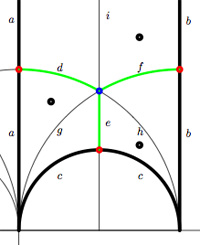$$\xymatrix{& & \rho \ar[lld]_d \ar[ld]^f \ar[rd]^e & \\ i \ar[rrd]_a & i+1 \ar[rd]^b & & \omega \ar[ld]^c \\ & & 0 \ar[uu]^h \ar@/^/[uu]^g \ar@/_/[uu]_i &}$$

In this case the super-potential coming from the triangulation is

$\Phi = -aid+agd-cge+che-bhf+bif$

and therefore we have a noncommutative algebra $\mathcal{U}(Q,\Phi)$ associated to this index 3 subgroup. Contrary to what I believed at the start of this series, the algebras one obtains in this way from dessins d’enfants are far from being Calabi-Yau (in whatever definition). For example, using a GAP-program written by Raf Bocklandt Ive checked that the growth rate of the above algebra is similar to that of $\mathbb{C}[x]$, so in this case $\mathcal{U}(Q,\Phi)$ can be viewed as a noncommutative curve (with singularities).

However, this is not the case for all such algebras. For example, the vacualgebra associated to the second index three subgroup (whose fundamental domain and quiver were depicted at the end of this post) has growth rate similar to that of $\mathbb{C} \langle x,y \rangle$…

I have an outlandish conjecture about the growth-behavior of all algebras $\mathcal{U}(Q,\Phi)$ coming from dessins d’enfants : the algebra sees what the monodromy representation of the dessin sees of the modular group (or of the third braid group).
I can make this more precise, but perhaps it is wiser to calculate one or two further examples…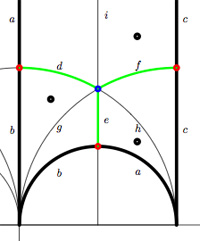We have associated to a subgroup of the modular group $PSL_2(\mathbb{Z})$ a quiver (that is, an oriented graph). For example, one verifies that the fundamental domain of the subgroup $\Gamma_0(2)$ (an index 3 subgroup) is depicted on the right by the region between the thick lines with the identification of edges as indicated. The associated quiver is then

$\xymatrix{i \ar[rr]^a \ar[dd]^b & & 1 \ar@/^/[ld]^h \ar@/_/[ld]_i \\ & \rho \ar@/^/[lu]^d \ar@/_/[lu]_e \ar[rd]^f & \\ 0 \ar[ru]^g & & i+1 \ar[uu]^c}$

The corresponding “dessin d’enfant” are the green edges in the picture. But, the red dot on the left boundary is identied with the red dot on the lower circular boundary, so the dessin of the modular subgroup $\Gamma_0(2)$ is

$\xymatrix{| \ar@{-}[r] & \bullet \ar@{-}@/^8ex/[r] \ar@{-}@/_8ex/[r] & -}$

Here, the three red dots (all of them even points in the Dedekind tessellation) give (after the identification) the two points indicated by a $\mid$ whereas the blue dot (an odd point in the tessellation) is depicted by a $\bullet$. There is another ‘quiver-like’ picture associated to this dessin, a quilt of the modular subgroup $\Gamma_0(2)$ as studied by John Conway and Tim Hsu.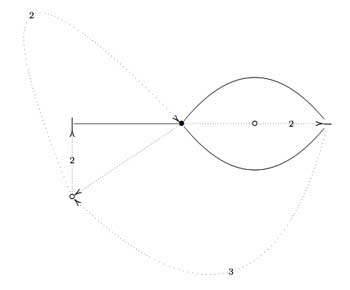On the left, a quilt-diagram copied from Hsu’s book Quilts : central extensions, braid actions, and finite groups, exercise 3.3.9. This ‘quiver’ has also 5 vertices and 7 arrows as our quiver above, so is there a connection?

A quilt is a gadget to study transitive permutation representations of the braid group $B_3$ (rather than its quotient, the modular group $PSL_2(\mathbb{Z}) = B_3/\langle Z \rangle$ where $\langle Z \rangle$ is the cyclic center of $B_3$. The $Z$-stabilizer subgroup of all elements in a transitive permutation representation of $B_3$ is the same and hence of the form $\langle Z^M \rangle$ where M is called the modulus of the representation. The arrow-data of a quilt, that is the direction of certain edges and their labeling with numbers from $\mathbb{Z}/M \mathbb{Z}$ (which have to satisfy some requirements, the flow rules, but more about that another time) encode the Z-action on the permutation representation. The dimension of the representation is $M \times k$ where $k$ is the number of half-edges in the dessin. In the above example, the modulus is 5 and the dessin has 3 (half)edges, so it depicts a 15-dimensional permutation representation of $B_3$.

If we forget the Z-action (that is, the arrow information), we get a permutation representation of the modular group (that is a dessin). So, if we delete the labels and directions on the edges we get what Hsu calls a modular quilt, that is, a picture consisting of thick edges (the dessin) together with dotted edges which are called the seams of the modular quilt. The modular quilt is merely another way to depict a fundamental domain of the corresponding subgroup of the modular group. For the above example, we have the indicated correspondences between the fundamental domain of $\Gamma_0(2)$ in the upper half-plane (on the left) and as a modular quilt (on the right)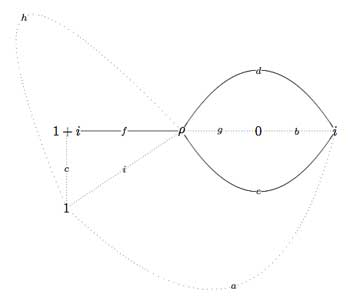That is, we can also get our quiver (or its opposite quiver) from the modular quilt by fixing the orientation of one 2-cell. For example, if we fix the orientation of the 2-cell $\vec{fch}$ we get our quiver back from the modular quilt$\xymatrix{i \ar[rr]^a \ar[dd]^b & & 1 \ar@/^/[ld]^h \ar@/_/[ld]_i \\ & \rho \ar@/^/[lu]^d \ar@/_/[lu]_e \ar[rd]^f & \\ 0 \ar[ru]^g & & i+1 \ar[uu]^c}$

This shows that the quiver (or its opposite) associated to a (conjugacy class of a) subgroup of $PSL_2(\mathbb{Z})$ does not depend on the choice of embedding of the dessin (or associated cuboid tree diagram) in the upper half-plane. For, one can get the modular quilt from the dessin by adding one extra vertex for every connected component of the complement of the dessin (in the example, the two vertices corresponding to 0 and 1) and drawing a triangulation from them (the dotted lines or ‘seams’).

This is how my attention was drawn to what I have since termed
anabelian algebraic geometry, whose starting point was exactly a study
(limited for the moment to characteristic zero) of the action of absolute
Galois groups (particularly the groups $Gal(\overline{K}/K)$, where K is an extension of finite type of the prime field) on (profinite) geometric fundamental
groups of algebraic varieties (defined over K), and more particularly (breaking with a well-established tradition) fundamental groups which are very far
from abelian groups (and which for this reason I call anabelian). Among
these groups, and very close to the group $\hat{\pi}_{0,3}$ , there is the profinite compactification of the modular group $SL_2(\mathbb{Z})$, whose quotient by its centre
$\{ \pm 1 \}$ contains the former as congruence subgroup mod 2, and can also be
interpreted as an oriented cartographic group, namely the one classifying triangulated oriented maps (i.e. those whose faces are all triangles or
monogons).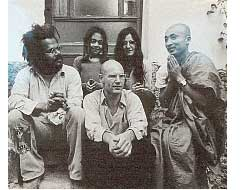The above text is taken from Alexander Grothendieck‘s visionary text Sketch of a Programme. He was interested in the permutation representations of the modular group $\Gamma = PSL_2(\mathbb{Z})$ as they correspond via Belyi-maps and his own notion of dessins d’enfants to smooth projective curves defined over $\overline{\mathbb{Q}}$. One can now study the action of the absolute Galois group $Gal(\overline{\mathbb{Q}}/\mathbb{Q})$ on these curves and their associated dessins. Because every permutation representation of $\Gamma$ factors over a finite quotient this gives an action of the absolute Galois group as automorphisms on the profinite compactification

$\hat{\Gamma} = \underset{\leftarrow}{lim}~\Gamma/N$

where the limit is taken over all finite index normal subgroups $N \triangleleft PSL_2(\mathbb{Z})$. In this way one realizes the absolute Galois group as a subgroup of the outer automorphism group of the profinite group $\hat{\Gamma}$. As a profinite group is a compact topological group one should study its continuous finite dimensional representations which are precisely those factoring through a finite quotient. In the case of $\hat{\Gamma}$ the simple continuous representations $\mathbf{simp}_c~\hat{\Gamma}$ are precisely the components of the permutation representations of the modular group. So in a sense, anabelian geometry is the study of these continuous simples together wirth the action of the absolute Galois group on it.

In noncommutative geometry we are interested in a related representation theoretic problem. We would love to know the simple finite dimensional representations $\mathbf{simp}~\Gamma$ of the modular group as this would give us all simples of the three string braid group $B_3$. So a natural question presents itself : how are these two ‘geometrical’ objects $\mathbf{simp}_c~\hat{\Gamma}$ (anabelian) and $\mathbf{simp}~\Gamma$ (noncommutative) related and can we use one to get information about the other?

This is all rather vague so far, so let us work out a trivial case to get some intuition. Consider the profinite completion of the infinite Abelian group

$\hat{\mathbb{Z}} = \underset{\leftarrow}{lim}~\mathbb{Z}/n\mathbb{Z} = \prod_p \hat{\mathbb{Z}}_p$

As all simple representations of an Abelian group are one-dimensional and because all continuous ones factor through a finite quotient $\mathbb{Z}/n\mathbb{Z}$ we see that in this case

$\mathbf{simp}_c~\hat{\mathbb{Z}} = \mu_{\infty}$

is the set of all roots of unity. On the other hand, the simple representations of $\mathbb{Z}$ are also one-dimensional and are determined by the image of the generator so

$\mathbf{simp}~\mathbb{Z} = \mathbb{C} – { 0 } = \mathbb{C}^*$

Clearly we have an embedding $\mu_{\infty} \subset \mathbb{C}^*$ and the roots of unity are even dense in the Zariski topology. This might look a bit strange at first because clearly all roots of unity lie on the unit circle which ‘should be’ their closure in the complex plane, but that’s because we have a real-analytic intuition. Remember that the Zariski topology of $\mathbb{C}^*$ is just the cofinite topology, so any closed set containing the infinitely many roots of unity should be the whole space!

Let me give a pedantic alternative proof of this (but one which makes it almost trivial that a similar result should be true for most profinite completions…). If $c$ is the generator of $\mathbb{Z}$ then the different conjugacy classes are precisely the singletons $c^n$. Now suppose that there is a polynomial $a_0+a_1x+\ldots+a_mx^m$ vanishing on all the continuous simples of $\hat{\mathbb{Z}}$ then this means that the dimensions of the character-spaces of all finite quotients $\mathbb{Z}/n\mathbb{Z}$ should be bounded by $m$ (for consider $x$ as the character of $c$), which is clearly absurd.

Hence, whenever we have a finitely generated group $G$ for which there is no bound on the number of irreducibles for finite quotients, then morally the continuous simple space for the profinite completion

$\mathbf{simp}_c~\hat{G} \subset \mathbf{simp}~G$

should be dense in the Zariski topology on the noncommutative space of simple finite dimensional representations of $G$. In particular, this should be the case for the modular group $PSL_2(\mathbb{Z})$.

There is just one tiny problem : unlike the case of $\mathbb{Z}$ for which this space is an ordinary (ie. commutative) affine variety $\mathbb{C}^*$, what do we mean by the “Zariski topology” on the noncommutative space $\mathbf{simp}~PSL_2(\mathbb{Z})$ ? Next time we will clarify what this might be and show that indeed in this case the subset

$\mathbf{simp}_c~\hat{\Gamma} \subset \mathbf{simp}~\Gamma$

will be a Zariski closed subset!

Hexagons keep on popping up in the representation theory of the modular group and its close associates. We have seen before that singularities in 2-dimensional representation varieties of the three string braid group $B_3$ are ‘clanned together’ in hexagons and last time Ive mentioned (in passing) that the representation theory of the modular group is controlled by the double quiver of the extended Dynkin diagram $\tilde{A_5}$, which is an hexagon…

Today we’re off to find representations of the extended modular group $\tilde{\Gamma} = PGL_2(\mathbb{Z})$, which is obtained by adding to the modular group (see this post for a proof of generation)

$\Gamma = \langle U=\begin{bmatrix} 0 & -1 \\\ 1 & 0 \end{bmatrix},V=\begin{bmatrix} 0 & 1 \\\ -1 & 1 \end{bmatrix} \rangle$ the matrix $R=\begin{bmatrix} 0 & 1 \\\ 1 & 0 \end{bmatrix}$

In terms of generators and relations, one easily verfifies that

$\tilde{\Gamma} = \langle~U,V,R~|~U^2=R^2=V^3=(RU)^2=(RV)^2=1~\rangle$

and therefore $\tilde{\Gamma}$ is the amalgamated free product of the dihedral groups $D_2$ and $D_3$ over their common subgroup $C_2 = \langle~R~\rangle$, that is

$\tilde{\Gamma} = \langle U,R | U^2=R^2=(RU)^2=1 \rangle \ast_{\langle R | R^2=1 \rangle} \langle V,R | V^3=R^2=(RV)^2=1 \rangle = D_2 \ast_{C_2} D_3$

From this description it is easy to find all n-dimensional $\tilde{\Gamma}$-representations $V$ and relate them to quiver-representations. $D_2 = C_2 \times C_2$ and hence has 4 1-dimensonal simples $S_1,S_2,S_3,S_4$. Restricting $V\downarrow_{D_2}$ to the subgroup $D_2$ it decomposes as

$V \downarrow_{D_2} \simeq S_1^{\oplus a_1} \oplus S_2^{\oplus a_2} \oplus S_3^{\oplus a_3} \oplus S_4^{\oplus a_4}$ with $a_1+a_2+a_3+a_4=n$

Similarly, because $D_3=S_3$ has two one-dimensional representations $T,S$ (the trivial and the sign representation) and one simple 2-dimensional representation $W$, restricting $V$ to this subgroup gives a decomposition

$V \downarrow_{D_3} \simeq T^{b_1} \oplus S^{\oplus b_2} \oplus W^{\oplus b_3}$, this time with $b_1+b_2+2b_3=n$

Restricting both decompositions further down to the common subgroup $C_2$ one obtains a $C_2$-isomorphism $V \downarrow_{D_2} \rightarrow^{\phi} V \downarrow_{D_3}$ which implies also that the above numbers must be chosen such that $a_1+a_3=b_1+b_3$ and $a_2+a_4=b_2+b_3$. We can summarize all this info about $V$ in a representation of the quiver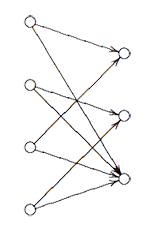Here, the vertex spaces on the left are the iso-typical factors of $V \downarrow_{D_2}$ and those on the right those of $V \downarrow_{D_3}$ and the arrows give the block-components of the $C_2$-isomorphism $\phi$. The nice things is that one can also reverse this process to get all $\tilde{\Gamma}$-representations from $\theta$-semistable representations of this quiver (having the additional condition that the square matrix made of the arrows is invertible) and isomorphisms of group-representation correspond to those of quiver-representations!

This proves that for all n the varieties of n-dimensional representations $\mathbf{rep}_n~\tilde{\Gamma}$ are smooth (but have several components corresponding to the different dimension vectors $~(a_1,a_2,a_3,a_4;b_1,b_2,b_3)$ such that $\sum a_i = n = b_1+b_2+2b_3$.

The basic principle of _M-geometry_ is that a lot of the representation theory follows from the ‘clan’ (see this post) determined by the simples of smallest dimensions. In the case of the extended modular group $\tilde{\Gamma}$ it follows that there are exactly 4 one-dimensional simples and exactly 4 2-dimensional simples, corresponding to the dimension vectors

$\begin{cases} a=(0,0,0,1;0,1,0) \\\ b=(0,1,0,0;0,1,0) \\\ c=(1,0,0,0;1,0,0) \\\ d=(0,0,1,0;1,0,0) \end{cases}$ resp. $\begin{cases} e=(0,1,1,0;0,0,1) \\\ f=(1,0,0,1;0,0,1) \\\ g=(0,0,1,1;0,0,1) \\\ h=(1,1,0,0;0,0,1) \end{cases}$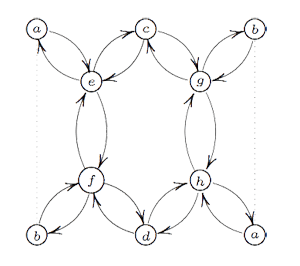If one calculates the ‘clan’ of these 8 simples one obtains the double quiver of the graph on the left. Note that a and b appear twice, so one should glue the left and right hand sides together as a Moebius-strip. That is, the clan determining the representation theory of the extended modular group is a Moebius strip made of two hexagons!

However, one should not focuss too much on the hexagons (that is, the extended Dynkin diagram $\tilde{A_5}$) here. The two ‘backbones’ (e–f and g–h) have their vertices corresponding to 2-dimensional simples whereas the topand bottom vertices correspond to one-dimensional simples. Hence, the correct way to look at this clan is as two copies of the double quiver of the extended Dynkin diagram $\tilde{D_5}$ glued over their leaf vertices to form a Moebius strip. Remark that the components of the sotropic root of $\tilde{D_5}$ give the dimensions of the corresponding $\tilde{\Gamma}$ simples.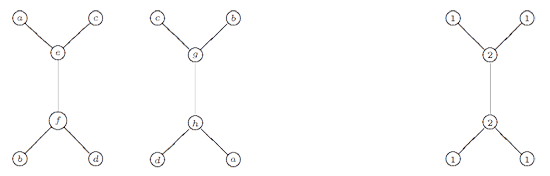The remarkable ubiquity of (extended) Dynkins never ceases to amaze!